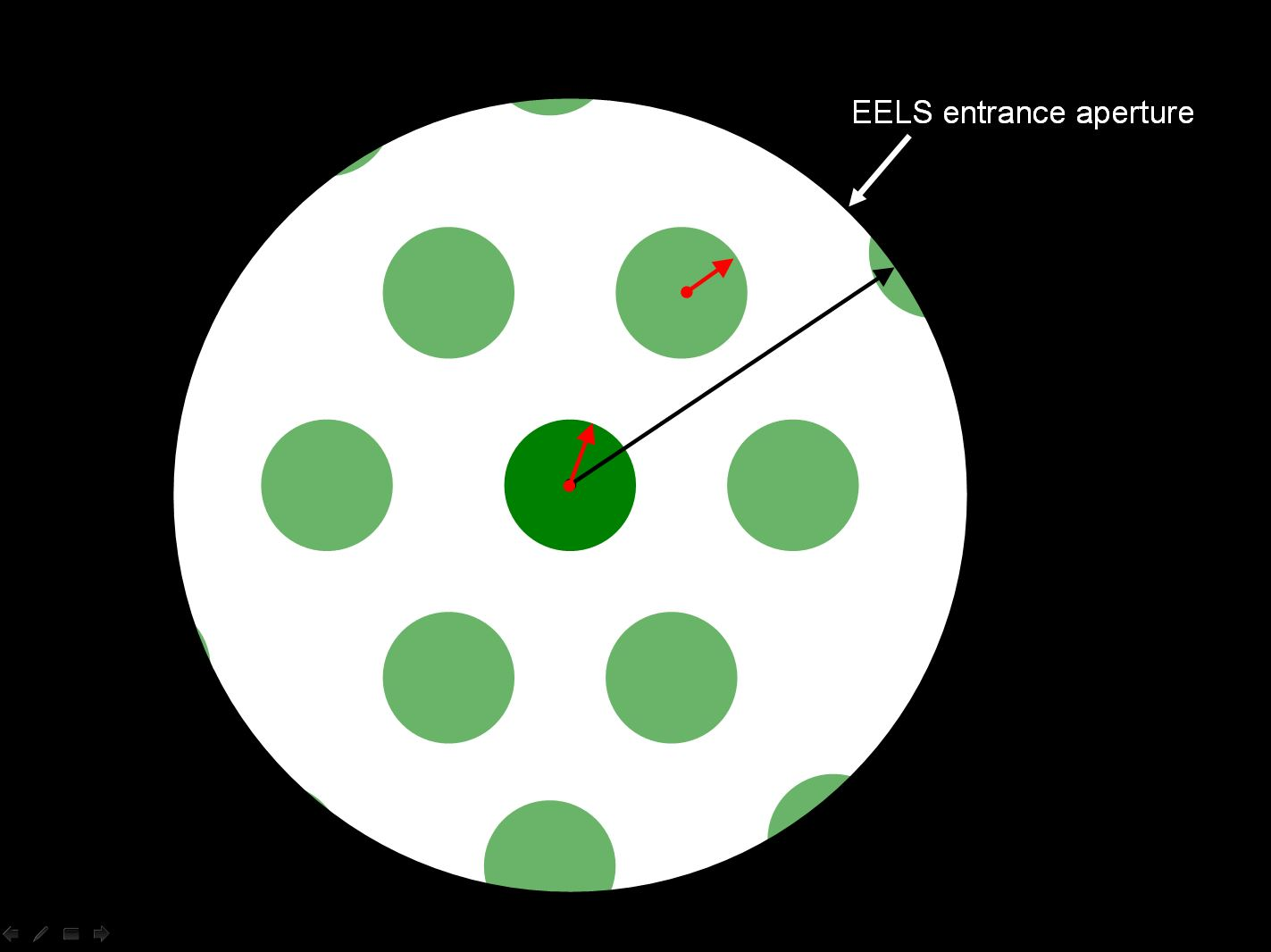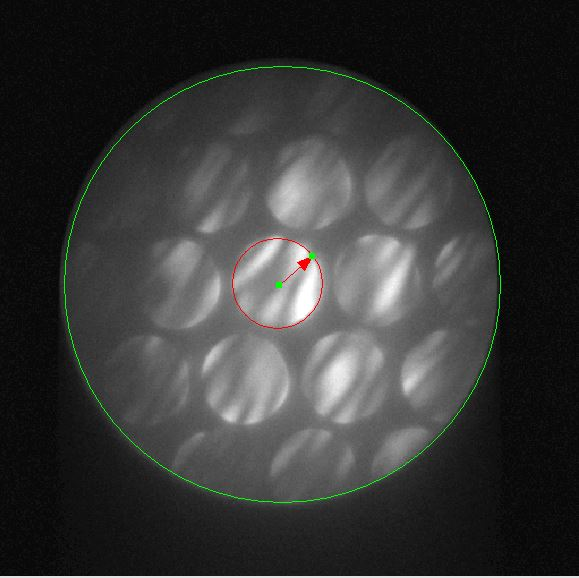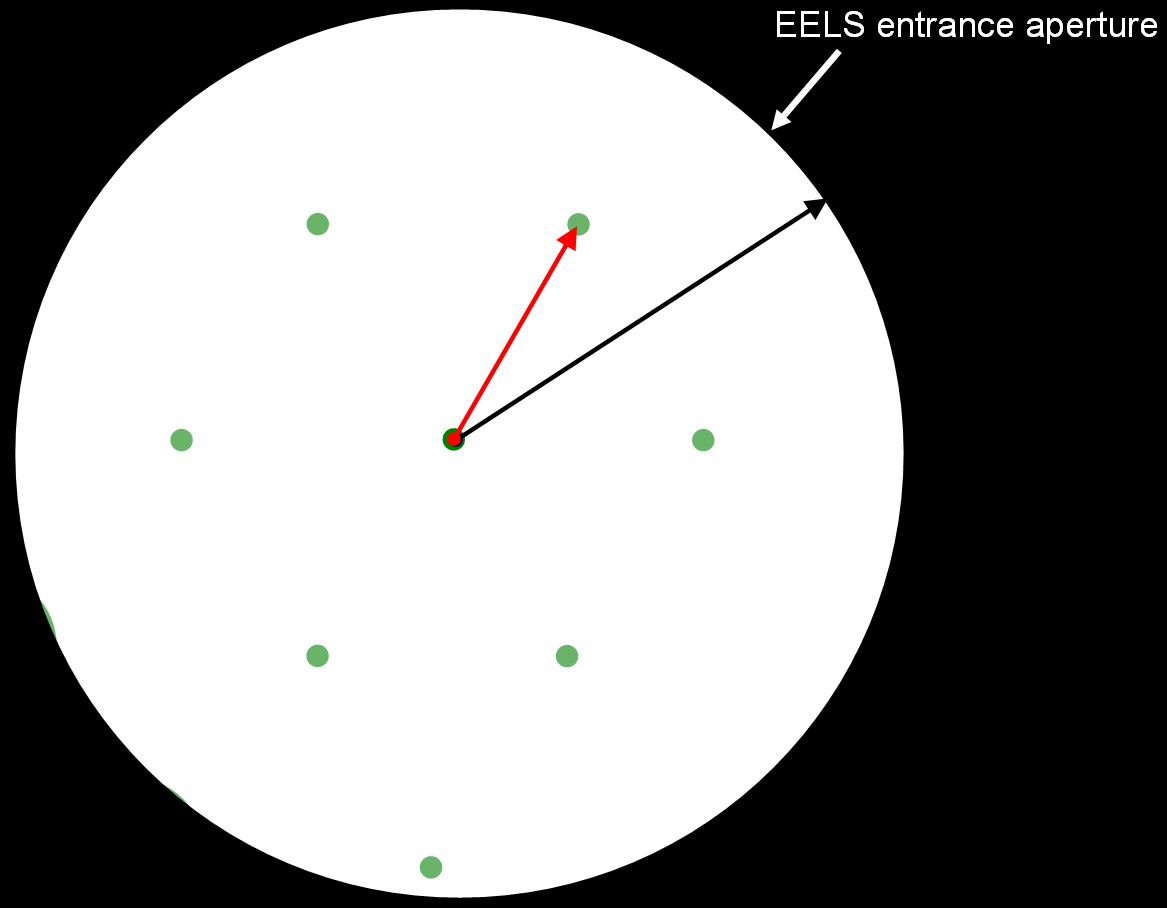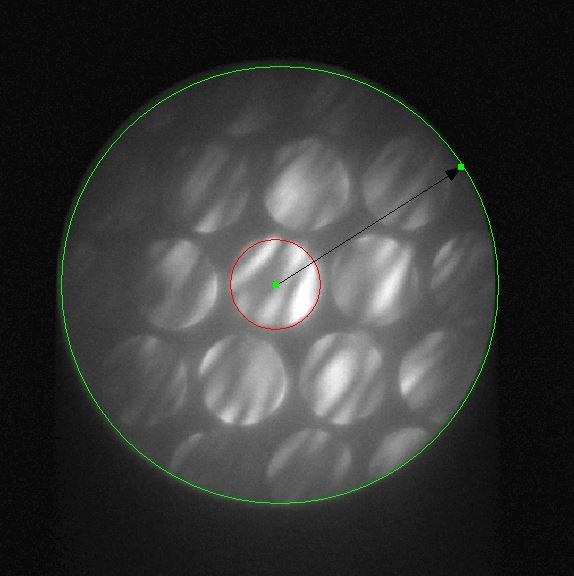EELS Collection Angles in Diffraction and STEM Modes,
and Collection Angle in EFTEM Mode, and Their Measurements
- Practical Electron Microscopy and Database -
- An Online Book -

https://www.globalsino.com/EM/

=================================================================================

In STEM and diffraction modes, the collection angle is determined by the selected camera length and the physical size of the entrance aperture. For dedicated STEM, the calculation of collection angle is very simple as shown in Figure 4939a below.Figure 4939a. Electron beam path in a dedicated STEM.

In this type of microscope no lenses exist between the specimen and the spectrometer collection (entrance) aperture. The collection semiangle is given by,

β ≈ R/L -------------------------------------------------- 

here, R is the radius of the EELS spectrometer entrance aperture and L the distance from the specimen to the aperture. For instance, L is ~ 200 mm and R is 2.5 mm, then β is 12.5 mrads.

If an objective aperture is inserted, the objective aperture is the collection aperture in both TEM imaging and EELS/GIF modes. If no objective aperture is inserted, the entrance aperture of EELS/GIF is the collection aperture.

In normal TEM diffraction and STEM modes, the situation is a little more complicated than in TEM imaging mode. We can extract the collection angles of EELS by the same way for both TEM diffraction and STEM modes. In those cases, we focus the spectrometer on an image of the specimen; so we see a DP (diffraction pattern) on the screen and also in the plane of the spectrometer-entrance aperture (e.g. entrance aperture of GIF camera made by Gatan). The collection semi-angle can be extracted as seen in the section of Collection Angle in TEM Diffraction and STEM Modes.

The schematics in Figure 4939b shows the electron optical column in a modern analytical electron microscope operated in STEM mode, indicating the projector lens controlling detector collection angle.Figure 4939b. Schematics of the electron optical column in a modern
analytical electron microscope operated in STEM mode.

In STEMs, the projector system normally consists of not less than two lenses that provide flexibility in choosing different camera lengths and  collection angles for imaging and spectroscopy.

The procedure of measuring the collection semi-angle of EELS in STEM mode is very simple:
i) Align the microscope in STEM mode.
ii) Align the Ronchigram to the center of the screen or camera in STEM mode.
iii) Obtain the convergence semi-angle in STEM mode. For instance, the measured convergence semi-angle of the red circle (the transmitted disc) in Figure 4939c (b) is 7.4 mrad and 80 pixels in the figure, so that the angle per pixel in the figure is 7.4/80 = 0.0925 mrad/pixel.
iii) Insert an entrance aperture (the black and green circles in Figure 4939) of the EELS system.
iv) Measure the pixel size of the black arrow (the radius of the entrance aperture) in Figure 4939c (d), which is 193 pixels.
v) Calculate the collection semi-angle for this specific entrance aperture, for instance, here is193 pixels * 0.0925 mrad/pixel = 16.8 mrad.

In electron diffraction mode, the procedure of measuring the collection semi-angle of EELS is:
i) Align the microscope in diffraction mode.
ii) Align the transmitted beam of the diffraction pattern to the center of the screen or camera.
iii) Obtain the angle of a known diffraction spot in pixel.
iv) Figure out the diffracted angle of this specific diffraction spot.
iv) Calculate the angle per pixel by using the numbers obtained in Steps iii and iv.
iii) Insert an entrance aperture (the black circles in Figure 4939 (c)) of the EELS system.
iv) Measure the pixel size of the black arrow (the radius of the entrance aperture) in Figure 4939c (c).
v) Calculate the collection semi-angle for this specific entrance aperture.

In EFTEM mode, the collection angle is defined by the size of the objective aperture and can be calculated with a diffraction pattern of a known sample, similar to the evaluation method in electron diffraction mode above.(a) (b)(c) (d)
 Figure 4939c. Measurement of collection semi-angle of EELS on GIF camera in both STEM and diffraction modes: (a) Schematic of the measurement in STEM mode, (b) and (d) A real picture of entrance aperture and beam-discs in STEM mode, and (c) Schematic of the measurement in electron diffraction mode.

=================================================================================